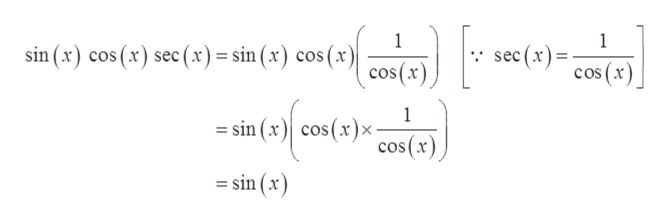# Use the fundamental identities to fully simplify the expression.sin(x) cos(x) sec(x)

Question
1 views

Use the fundamental identities to fully simplify the expression.

sin(x) cos(x) sec(x)
check_circle

Step 1

Given:

Step 2

Now Simplifying the gi...help_outlineImage Transcriptionclose1 sin (x) cos (x) sec (x)= sin (x) cos (x)| cos(x) 1 = cos (x sec (x) 1 =sin (x) cos(x)x - cos(x =sin (x) fullscreen

### Want to see the full answer?

See Solution

#### Want to see this answer and more?

Solutions are written by subject experts who are available 24/7. Questions are typically answered within 1 hour.*

See Solution
*Response times may vary by subject and question.
Tagged in

### Trigonometric Ratios